Home > CCA2 > Chapter 8 > Lesson 8.1.2 > Problem8-44

8-44.
1. For each equation, find two solutions 0° ≤ θ < 360°, which make the equation true. You should not need a calculator. Homework Help ✎1. sin θ =2. tan θ =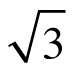3. cos θ =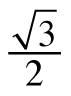4. sin θ = −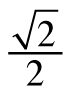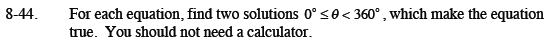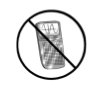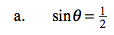Draw and label a unit circle for each.

30° and 150°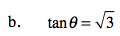60°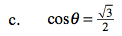30°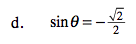225°